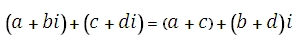# XLeratorDB/engineering Online Documentation

Updated: 27 August 2010
Use IMADD to return the sum of 2 complex numbers in x + yi or x + yj text format. The sum of 2 complex numbers is computed as:Syntax
<@inumber1, nvarchar(4000),>
,<@inumber2, nvarchar(4000),>)
Arguments
@inumber1
is the first value used in evaluating the function. @inumber1 is an expression of type varchar or of a type that can be implicitly converted to varchar and must be in the format x + yi or x + yj, where x is a real number that can be converted to float and yi (or yj) is an imaginary number, where the y part can be converted to float.
@inumber2
is the second value used in evaluating the function. @inumber2 is an expression of type varchar or of a type that can be implicitly converted to varchar and must be in the format x + yi or x + yj, where x is a real number that can be converted to float and yi (or yj) is an imaginary number, where the y part can be converted to float.
Return Types
varchar(4000)
Remarks
·         Use COMPLEX to convert real and imaginary coefficients into a complex number
Examples

select

This produces the following result
-------------------------
13-i

(1 row(s) affected)

### SupportCopyright 2008-2023 Westclintech LLC         Privacy Policy        Terms of Service